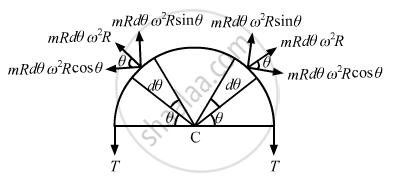Advertisement Remove all ads

# A Circular Loop of String Rotates About Its Axis on a Frictionless Horizontal Place at a Uniform Rate So that the Tangential Speed of Any Particle of the String is - Physics

Sum

A circular loop of string rotates about its axis on a frictionless horizontal place at a uniform rate so that the tangential speed of any particle of the string is ν.  If a small transverse disturbance is produced at a point of the loop, with what speed (relative to the string) will this disturbance travel on the string?

Advertisement Remove all ads

#### Solution

Let,
V = Linear velocity of the string
m = Mass per unit length of the the string.
R = Radius of the loop
ω = Angular velocityConsider one half of the string, as shown in the figure.
The half loop experiences centrifugal force at every point (away from the centre) balanced by tension 2T.
Consider an element of angular part dθ at angle θ.
So,
Length of the element
$= Rd\theta, mass = mRd\theta$
Centrifugal force experienced by the element
$= \left( mRd\theta \right) \omega^2 R$
Resolving the centrifugal force into rectangular components,
Since the horizontal components cancel each other, the net force on the two symmetric elements is given as
$dF = 2m R^2 d\theta \omega^2 sin\theta$

$Total force, F = \int_0^\pi/2 2m R^2 \omega^2 \sin\theta d\theta$

$= 2m R^2 \omega^2 \left[ - \cos \theta \right]$

$= 2m R^2 \omega^2$

$And,$

$2T = 2m R^2 \omega^2$

$\Rightarrow T = m R^2 \omega^2$

Velocity of the transverse vibration is given as

$V' = \sqrt{\left( \frac{T}{m} \right)}$

$V' = \sqrt{\left( \frac{m R^2 \omega^2}{m} \right)} = \omega R$
Linear velocity of the string, V = $\omega R$
∴ Speed of the disturbance, V' =  V

Is there an error in this question or solution?
Advertisement Remove all ads

#### APPEARS IN

HC Verma Class 11, 12 Concepts of Physics 1
Chapter 15 Wave Motion and Waves on a String
Q 25 | Page 325
Advertisement Remove all ads
Advertisement Remove all ads
Share
Notifications

View all notifications

Forgot password?